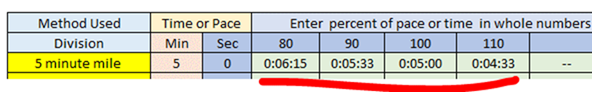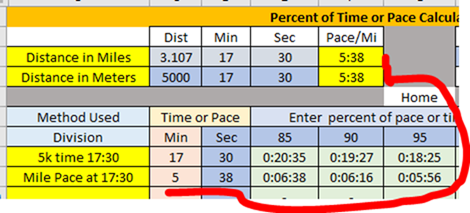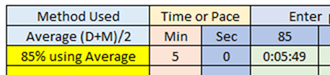﻿ PacePercents
 Here's how this works... (To get to the tables click on the Percents tab above.) Many training plans use percentages of another value whether it's a percentage of a PR, vVO2max, Date Pace for some distance, etc. This calculator has one purpose and that's to compute a percentage of pace or time for an initial value. For example, the table below shows 80%, 90%, 100%, and 110% of 5 minutes. This could be a time of 5 minutes over some distance or a pace of 5 minutes per mile.What are 85%, 90\$, and 95%  of a 5000-meter time of 17:30?  They're 20:35, 19:27, and 18:25. What are the mile paces associated with that? They're 6:38, 6:16, and 5:56. For the mile paces I used the pace tool at the top left of the screen to get the mile pace for 5000 meters. For demo purposes I also used the mile equivalent of 5k... 3.107 There's also a conversion tool at the upper right of the screen that converts meters to miles and vice versa.OK, but what's the "Method Used" Dropdown?As noted above the most common way of assigning effort or pace for a certain type of workout is to describe it in terms of percent of some known value. The problem here is just what is 85% of a time or pace. Take a 5-minute mile for example. Some, if not most, would argue that you divide 5 minutes by .85 for 5:53. Others would say that you should multiply 5 minutes by 1.15 for 5:45. Which is correct? If we were trying to ascertain how much is 85 percent of 10 inches it's obvious that we'd multiply by .85 for 8.5 inches. Dividing by 1.15 would give 8.7 inches which is obviously incorrect. It's different for pace or time because multiplying or dividing by .85 gives us a slower time (larger number) than the smaller value obtained when considering a percent of length. Coach John Davis has a popular blog that investigates this issue at RunningWritings.com. He points out that the multiplication method yields a linear progression of differences in Speed, while the Division method yields a linear progression of differences in Pace. In each case the "Division Method" yields a more conservative (slower) value than the "Multiplication Method." Since most of the coaches whose methodology I subscribe to use the division method (Scott Christenson, Dr. Joe Vigil, Dr. Jeff Messer to name a few) I use the "Division Method," that's the default for these tables. However, because many  use the more aggressive "Multiplication Method," I included a drop-down that will let you select either method, as well as an average between the two.Please email any errors, changes, or suggestions to George Green : Plugh@CrownCity.com Home
 Percent of Time or Pace Calculator Dist Min Sec Pace/Mi Miles To Meters Meters To Miles Distance in Miles Distance in Meters Home Method Used Time or Pace Enter  percent of pace or time  in whole numbers below eg 75% = 75 Division Multiplication Average (D+M)/2 Min Sec -------------------------- ---------- '---------- ------------ ------------ '------------ '------------ '------------ '------------ '------------